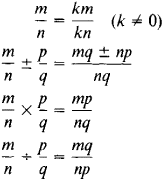# rational number

(redirected from Field of rationals)
Also found in: Dictionary, Thesaurus, Financial.

## rational number:

see numbernumber,
entity describing the magnitude or position of a mathematical object or extensions of these concepts. The Natural Numbers

Cardinal numbers describe the size of a collection of objects; two such collections have the same (cardinal) number of objects if their
..... Click the link for more information.
.
The Columbia Electronic Encyclopedia™ Copyright © 2013, Columbia University Press. Licensed from Columbia University Press. All rights reserved. www.cc.columbia.edu/cu/cup/
The following article is from The Great Soviet Encyclopedia (1979). It might be outdated or ideologically biased.

## Rational Number

a number that can be expressed in the form of a fraction m/n, where m and η are integers and n ≠ 0. Since an integer m can be expressed as m/1, all integers are rational numbers. The operations of addition, subtraction, multiplication, and division (by nonzero divisors) can always be performed in the domain of rational numbers; thus, the rational numbers form a field. The basic rules of the operations over the rational numbers are given by the formulasRational numbers can also be represented in the form of finite decimals or infinite periodic decimals. Every irrational number can be included between two rational numbers, the difference between which can be made arbitrarily small.

The Great Soviet Encyclopedia, 3rd Edition (1970-1979). © 2010 The Gale Group, Inc. All rights reserved.

## rational number

[′rash·ən·əl ′nəm·bər]
(mathematics)
A number which is the quotient of two integers.
McGraw-Hill Dictionary of Scientific & Technical Terms, 6E, Copyright © 2003 by The McGraw-Hill Companies, Inc.

## rational number

any real number of the form a/b, where a and b are integers and b is not zero, as 7 or 7/3
Collins Discovery Encyclopedia, 1st edition © HarperCollins Publishers 2005
Site: Follow: Share:
Open / Close